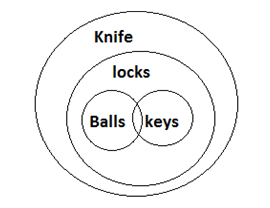# CMAT Logical Reasoning Practice Questions Set-3

CMAT Logical Reasoning Practice questions Set-3: This practice set has 10 questions with their answers and Explanatory answers covering topics from the areas of Logical Reasoning.

Dec 9, 2015 17:38 IST

CMAT Logical Reasoning Practice questions Set-3: This practice set has 10 questions with their answers and Explanatory answers covering topics from the areas of Logical Reasoning.

Directions (1 -4): Study the following information and answer the questions given below:

Eight persons A1, A2, A3, A4, A5, A6, A7, and A8 are sitting around a rectangular table in such a way that four persons sits on one side facing north and other four person are sitting on the opposite side of the first four persons and they are facing south (no one is sitting on the two other side of the table). So, in this arrangement each member sitting in a row faces another member of the opposite row.

A1 is facing north and A7 is sitting opposite to him and both of them are at the corner seats. A2 and A6 are sitting in the same row. A5 is sitting opposite to A2. A4 is sitting opposite to A6 and A5 is on the immediate right of A1.A8 is sitting on the corner seat on the immediate left of A6.

1. Who is sitting opposite to A3?

a) A2
b) A6
c) A7
d) A8

2. Who is sitting in between A2 and A8?

a) A6
b) A7
c) A5
d) A4

3. Who is sitting diagonally opposite to A7?

a) A1
b) A3
c) A5
d) A3

4. Who is sitting at the left of A6?

(a) 7
(b) 4
(c) 3
(d) 8

In each of the questions below consists of a question and two statements numbered I and II given below it. You have to decide whether the data provided in the statements are sufficient to answer the question. Read both the statements and

a) If the data in statement I alone are sufficient to answer the question, while the data in statement II alone are not sufficient to answer the question.
b) If the data in statement II alone are sufficient to answer the question, while the data in statement I alone are not sufficient to answer the question.
c) If the data either in statement I alone or in statement II alone are sufficient to answer the question.
d) If the data given in both statements I and II together are not sufficient to answer the question and.
e) If the data in both statements I and II together are necessary to answer the question.

5. How far is point A from point B. (All the points lie on a straight line)

I. Point C is exactly midway between points A and B. Point C is 5 Km towards west of point D.
II. Point B is 2 Kms towards the east of point D.

6. Pointing to a man in the Photograph Kabir said, ‘His son’s mother is the only daughter in law of my mother’.  Who is the person in photograph to Kabir?

a) Brother
b) Uncle
c) Father-in-law
d) None of these

Below is given a passage followed by several possible inferences which can be drawn from the facts stated in the passage. You have to examine each inference separately in the context of the passage and decide upon its degree of truth or falsity.

Relations between India and Sri Lanka have not just been reinforced during the visit of President Maithripala Sirisena but have also gained new direction and momentum. As Sri Lanka’s closest neighbour that has ethnic links to its most significant minority, India is a huge influence in the island nation’s political, economic, social and cultural consciousness, and its world view. The agreement on Co-operation in the Peaceful Uses of Nuclear Energy is the most significant strategic element to bilateral relations. Since 2010, Sri Lanka has wanted to utilise nuclear energy in industrial applications as well as in fields such as medicine and agriculture.

7. If India enters into Nuclear Agreement with Sri Lanka, it will improve the relationship between the two nations?

a) If inference is definitely true.
b) If inference is probably true.
c) If the data are inadequate.
d) If inference is probably false.
e) If the inference false.

8. Point Q is 5 meters north of point P. Point R is 5 meters west of point Q. Point S is 7 meters south of point R. Point T is 9 meters east of point S. In which of the following directions is point T with reference to point Q?

a) North east
b) South west
c) South east
d) North

Directions (9 – 10) In each of the questions below are given three statements followed by two conclusions numbered I and II. You have to take the given statements to be true even if they seem to be at variance with commonly known facts. Read all the conclusions and then decide which of the given conclusions logically follows from the given statements, disregarding commonly known facts. Give answer-

a) If only conclusion I follows.
b) If only conclusion II follows.
c) If either conclusion I or conclusion II follows.
d) If neither conclusion I nor conclusion II follows.
e) If both conclusions I and II follow.

9.

Statements: All pearls are stones. Some pearls are diamonds. No diamond is gem.

Conclusions:

I. All pearls, if they are diamonds, are also gem.
II. At least some diamonds being gem are a possibility.

10.

Statements: All balls are locks. Some balls are keys. All locks are knifes.

Conclusions:

I. Some balls are not knifes.
II. All locks being keys is a possibility.

1. (d), 2. (a), 3. (d), 4. (d), 5. (e), 6. (d), 7. (a), 8. (c), 9. (b), 10. (b)

Explanations:

Explanation 4:

By the information given we can have the following arrangement.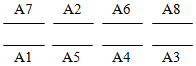Explanation 5: From both the statements.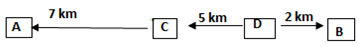Explanation 6: The person is Kabir himself.

Explanation 7: It can be clearly inferred from the passage that bilateral relations will be improve after the nuclear deal.

Explanation 8: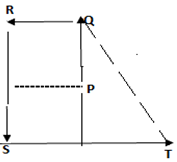Explanation 9: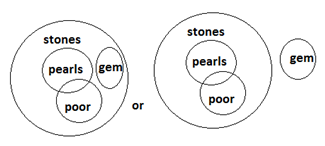Explanation 10: